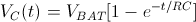What is electric charge? Electric charge is a fundamental physical property of matter. Electric charge can be positive or negative. Matter repels other matter of the same charge and attracts other matter having the opposite charge. The unit used for electric charge is a Coulomb [C]. While the exact nature of charge is still unknown at a fundamental level, it is generally accepted to represent a specific state of matter which cannot be explained at the current level of scientific knowledge. Electric charge is quantized, meaning that charge can only have discrete values. An elementary charge is denoted as e, and approximately equals 1.602·10-19 C. The electron bears a charge of -e and it is a negatively charged particle. In contrast, a proton is a positively charged particle, bearing a charge of +e. An intuitive way to understand the quantized nature of charge is to imagine an electrically neutral object as a box containing an equal number of protons (positive charges) and electrons (negative charges). Protons are fixed and cannot be taken out or added to the box. Since the number of protons and electrons is equal, the total sum of the electric charge inside the box is zero for electrically neutral objects. In order to make the object negatively charged, the only way to do so is to somehow add more electrons into the box. As electrons are indivisible particles, it is only possible to add an integer number of electrons – one cannot add half an electron into the box. As a result, the total charge of the object is N times the charge of a single electron, which equals -e·N, where N is an integer number. Similarly, in order to make an object positively charged, it is necessary to remove N electrons from the box and the [… read more]